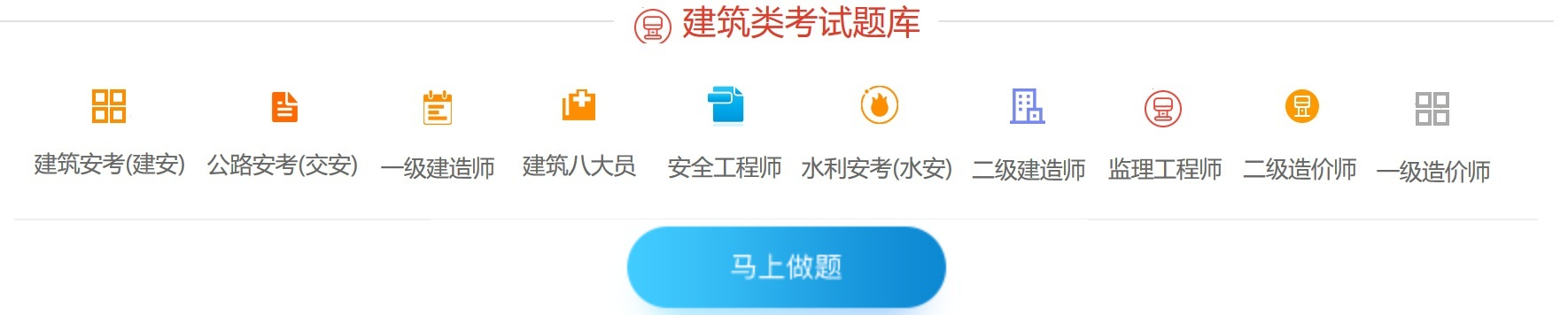# 2021版建筑材料员模拟题

[点击进入2021版建筑材料员模拟题]

A.395.1

B.386.3

C.420.4

D.370.9

A.不必调整

B.应调整

C.视需要调整

D.局部调整

A.合理选择路线

B.合理选择运输方式

C.采取直达运输

D.合理组织材料装载

A.18.96万元

B.20.95万元

C.20.28万元

D.21.47万元

A.5.0

B.9.5

C.4.75

D.2.36

A.保供.保退.保收

B.保供.保量.保进度

C.保质.保量.保进度

D.保质.保量.保维修

A.正面图

B.剖面图

C.局部投影图

D.断面图

A.房地产开发.工程设计.建筑施工

B.工程设计.建筑施工.工程监理

C.施工总承包.专业承包.劳务分包

D.工程总承包.施工承包.专业分包

A.供货方

B.储存能力

C.资源渠道

D.市场供求关系

A.水泥砂浆

B.石灰砂浆

C.水泥石灰混合砂浆

D.吸声砂浆

A.状态变化

B.物体产生变形

C.失去稳定

D.破坏效应

A.乙烯

B.聚氯乙烯

C.聚苯乙烯

D.酚醛树脂

A.20000

B.6000

C.8000

D.10000

A.计量检验

B.验收检验

C.使用检验

D.优良检验

A.强度提高，塑性增强

B.强度降低，塑性降低

C.强度降低，塑性增强

D.强度提高，塑性降低

A.弹性

B.塑性

C.强度

D.冷弯性能

A.质量

B.数量

C.工程量

D.资金损耗量

A.工程监理单位

B.建设单位

C.建设工程质量和安全生产监督机构

D.施工单位

A.浇筑地点

B.搅拌地点

C.实验室

D.办公室

A.项目经理

B.建造师

C.公司经理

D.工地负责人

A.λ1> λ2> λ3

B.λ1< λ2< λ3

C.λ1> λ2= λ3

D.λ1< λ2 = λ3

A.验收前准备.核对验收资料.检验实物.办理入库手续

B.验收前准备.检验实物.核对验收资料.办理入库手续

C.核对验收资料.验收前准备.验收实物.办理入库手续

D.核对验收资料.办理入库手续.验收前准备.检验实物

A.颈缩阶段

B.强化阶段

C.屈服阶段

D.弹性阶段

A.储存期限

B.过期失效

C.储存期限.过期失效

D.注意防潮.过期失效

A.P·S

B.P ·O

C.P ·P

D.P ·F

A.采取枝术措施，节约材料

B.加强材料管理，降低材料消耗

C.实行现场材料承包责任制，提高经济效益

D.实行材料浪费惩罚制度

E.实行材料节约奖励制度，提高节约材料的积极性材料装修装饰部分《多选题》

A.书面检验

B.外观检验

C.理化检验

D.无损检验

E.目测检验

A.聚乙烯醇水玻璃内墙涂料

B.聚乙烯醇缩甲醛类墙.地面涂料

C.丙烯酸醋

D.氯化橡胶

E.聚乙烯醇缩丁醛

A.掌握材料信息，选择价格相对便宜的厂家，节约成本

B.合理组织材料供应，确保施工正常进行

C.合理组织材料使用，减少材料损失

D.加强现场材料管理

E.材料进场后进行粗加工时要检查验收合格，方可使用

A.合同订购

B.自由选购

C.租赁

D.联合订货

A.最大粒径与颗粒级配

B.强度

C.粗细程度

D.有害杂质含量

A.物流形式

B.加工形式

C.提货形式

D.现货现购

E.签订合同形式

A.外观质量验收

B.内在化学成分验收

C.力学性能的验收

D.冷弯性能验收

A.抗压强度

B.抗折强度

C.抗剪强度

D.抗拉强度

E.干密度

A.满足混凝土强度要求

B.小于最大水灰比规定

C.大于最大水灰比规定

D.不考虑最大水灰比规定

A.抗压强度

B.抗折强度

C.堆积密度和细度

D.矿物组成和细度

A.行政方法

B.经济方法

C.招标投标法

D.法律方法

A.建材生产厂家因素

B.气候条件变化

C.材料市场需求变化

D.施工进度变化

A.合理的工艺损耗

B.非操作损耗

C.净用量

D.合理的管理损耗

A.高压水

B.黄沙

C.干粉灭火器

D.其他方式

A.1.5

B.2.0

C.3.0

D.10.0

A.专业队检查制度

B.现场检查制度

C.统计检查制度

D.定期检查制度

E.公司检查制度

A.同样的材料比质量

B.同样的质量比价格

C.同样的价格比运距

D.同样的价格比材料

A.HRB335

B.HRB400

C.HRB500

D.HRB235

E.HRB300

A.销售计划

B.材料供应计划

C.采购计划

D.运输计划

A.合理的工艺损耗

B.非操作损耗

C.净用量

D.合理管理损耗

A.企业管理人员

B.专业技术人员

C.技术装备

D.财务状况

A.运输条件

B.材料特性

C.需用单位的生产规模

D.材料价格

A.推销法

B.租赁法

C.费用承包法

D.实物承包法

A.材料采购核算

B.材料储备核算

C.材料供应核算

D.材料消耗核算

A.正确

B.错误

A.正确

B.错误

A.正确

B.错误

A.正确

B.错误

A.正确

B.错误

A.正确

B.错误

A.正确

B.错误

A.正确

B.错误

A.正确

B.错误

A.正确

B.错误

A.正确

B.错误

A.正确

B.错误

A.正确

B.错误

A.正确

B.错误

A.正确

B.错误

A.正确

B.错误

A.正确

B.错误

A.正确

B.错误

A.正确

B.错误

A.正确

B.错误